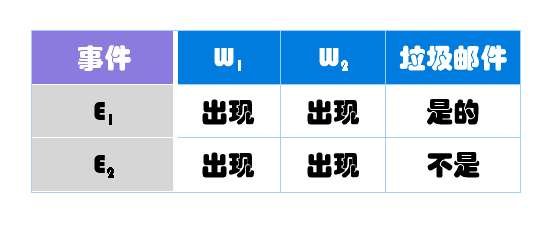# 贝叶斯推断及其互联网应用（二）：过滤垃圾邮件

========================================（接上文）

2002年，Paul Graham提出使用"贝叶斯推断"过滤垃圾邮件。他说，这样做的效果，好得不可思议。1000封垃圾邮件可以过滤掉995封，且没有一个误判。

"训练"过程很简单。首先，解析所有邮件，提取每一个词。然后，计算每个词语在正常邮件和垃圾邮件中的出现频率。比如，我们假定"sex"这个词，在4000封垃圾邮件中，有200封包含这个词，那么它的出现频率就是5%；而在4000封正常邮件中，只有2封包含这个词，那么出现频率就是0.05%。（【注释】如果某个词只出现在垃圾邮件中，Paul Graham就假定，它在正常邮件的出现频率是1%，反之亦然。这样做是为了避免概率为0。随着邮件数量的增加，计算结果会自动调整。）Paul Graham的做法是，选出这封信中P(S|W)最高的15个词，计算它们的联合概率。（【注释】如果有的词是第一次出现，无法计算P(S|W)，Paul Graham就假定这个值等于0.4。因为垃圾邮件用的往往都是某些固定的词语，所以如果你从来没见过某个词，它多半是一个正常的词。）（完）

## 留言（74条）

Mark 先！

P(E1)+P(E2)不等於1嗎？

1. P(E1)=P(S|W1)*P(S|W2)*P(S) (why?)
2. P=P(E1)/(P(E1)+P(E2)) 像楼上Allen说的，直觉是P(E1)+P(E2)=1

96年就有人发布了。

“将P(S|W1)记为P1，P(S|W1)记为P2，公式就变成”
“将P(S|W1)记为P1，P(S|W2)记为P2，公式就变成”

@3tgame：谢谢指出，已经更正了。

@水人：对，是经验值。好在可以根据新收的邮件不断调整。

@Allen：E1和E2是指后面三个事件同时发生，所以它们的和不等于1。

@hyh：Paul Graham发明的是现在这一套计算方法，大大提高了过滤效果，而不是发明用贝叶斯推断过滤邮件的概念。

`引用Bill的发言：`

1. P(E1)=P(S|W1)*P(S|W2)*P(S) (why?)

E1代表三个独立事件同时发生，因此E1的概率是后面三个概率的乘积。

`引用Bill的发言：`

2. P=P(E1)/(P(E1)+P(E2)) 像楼上Allen说的，直觉是P(E1)+P(E2)=1

P1应该是P（W1|S）吧？

http://science.solidot.org/article.pl?sid=11/08/06/147202

https://www.nytimes.com/2011/08/07/books/review/the-theory-that-would-not-die-by-sharon-bertsch-mcgrayne-book-review.html

P(S)=P(S|W1)XP(S|W2)/(P(S|W1)XP(S|W2)+(1-P(S|W1))X(1-P(S|W2)))

P(S)=P(S|W1)XP(W1)+P(S|W2)XP(W2)+P(S|W3)XP(W3)+……

`引用zc的发言：`

@ Mw3000:

Bayesian Inference:
P(A|B)=P(A)*P(B|A)/P(B)

@Bill:

P(S)=p(E1)/(P(E1)+P(E2))

`引用Bill的发言：`

1. P(E1)=P(S|W1)*P(S|W2)*P(S) (why?)
2. P=P(E1)/(P(E1)+P(E2)) 像楼上Allen说的，直觉是P(E1)+P(E2)=1

P(S|W1 W2) = P(W1 W2|S)P(S) / (P(W1 W2|S)P(S) + P(W1 W2|~S)P(~S))

W1,W2独立：P(W1 W2) = P(W1)P(W2), P(W1 W2|S) = P(W1|S)P(W2|S) (？)

= P1P2 / (P1P2 + (1-P1)(1-P2));

p(S|W1W2) = P(~S)P1P2/(P(~S)P1P2 + P(S)(1-P1)(1-P2))

ml-class.org.对比着这篇文章.收获蛮大..

P=P(E1)/(P(E1)+P(E2))

btw
P(S|W1 W2) = P(W1|S)P(W2|S)P(S) / (P(W1|S)P(W2|S)P(S) + P(W1|~S)P(W2|~S)P(~S))

`引用alex的发言：`

btw
P(S|W1 W2) = P(W1|S)P(W2|S)P(S) / (P(W1|S)P(W2|S)P(S) + P(W1|~S)P(W2|~S)P(~S))

Jin, 你的解释很赞~~解决了我的疑问！

P(S)=0.5这个假设会不会随着邮件的处理而做修正。但是我们的公式推导中用到了P(S)=0.5。

`引用gausszh的发言：`

P(S)=0.5这个假设会不会随着邮件的处理而做修正。但是我们的公式推导中用到了P(S)=0.5。

`引用Allan的发言：`

`引用gausszh的发言：`

`引用Jin的发言：`
…… W1,W2独立：P(W1 W2) = P(W1)P(W2), P(W1 W2|S) = P(W1|S)P(W2|S) (？) ……

http://www.mathpages.com/home/kmath267.htm

`引用waveacme的发言：`

http://www.mathpages.com/home/kmath267.htm

http://en.wikipedia.org/wiki/Cox's_theorem

Jin的解答中貌似也对这里不太明白。

P(S|W1)*P(S|W2)*P(S)这个用法是错误的。 （当然也可能是我理解不到位， 请指正）

P(S)*P(W1|S)*P(W2|S) / ( P(S)*P(W1|S)*P(W2|S)+P(~S)*P(W1|~S)*P(W2|~S) )
P(~S) 意为P(Not SPam)

=====================

`引用David_Li的发言：`

http://en.wikipedia.org/wiki/Cox's_theorem

Jin的解答中貌似也对这里不太明白。

P(S|W1)*P(S|W2)*P(S)这个用法是错误的。 （当然也可能是我理解不到位， 请指正）

P(S)*P(W1|S)*P(W2|S) / ( P(S)*P(W1|S)*P(W2|S)+P(~S)*P(W1|~S)*P(W2|~S) )
P(~S) 意为P(Not SPam)

=====================

1、15个W是怎么挑出来的？如果这个W在垃圾邮件和非垃圾邮件中的词频都很高，比如是“我”这个词，是否有一套筛选原则？
2、为什么公式推导到最后都没有用到 P(W/H)，但在一开始的训练集中又被要求计算了这个值。E2事件被定义为正常邮件，文中说P(E2)=(1-P(S|W1))(1-P(S|W2))(1-P(S))，那P(E2)=P(H|W1)P(H|W2)P(H)是否成立？

，基于Discovery Challenge 2006的一个垃圾邮件过滤竞赛，非常好用的方法，同时也用Logisitic Regression方法实现了一遍。

`引用49Degree的发言：`

∵P1=P(S|W1)，P2=P(S|W2), P = P(S|W1W2) = P(S∩W1∩W2)/P(W1W2);

∴P(S∩W1∩W2) = P(S∩W2)∩P(S∩W1) = P(S|W1)P(W1)P(S|W2)P(W2);

∴P(S|W1W2)=
P(S|W1)P(W1)P(S|W2)P(W2) / [(P(S|W1)P(W1)P(S|W2)P(W2) + P(~S|W1)P(W1)P(~S|W2)P(W2)]； ①
∵P1 = P(S|W1) = P(S∩W1)/P(W1),
P(~S∩W1)/P(W1) = P((1-S)∩W1)/P(W1) = [P(W1)-P(S∩W1)] / P(W1) = 1-P；②
②代入①得到
P = = P1P2 / (P1P2 + (1-P1)(1-P2));

"When new mail arrives, it is scanned into tokens, and the most interesting fifteen tokens, where interesting is measured by how far their spam probability is from a neutral .5, are used to calculate the probability that the mail is spam."

http://blog.csdn.net/hexinuaa/article/details/5596862

P(W|S)才是右边项，作为最大似然估计。

`引用Amble的发言：`

∵P1=P(S|W1)，P2=P(S|W2), P = P(S|W1W2) = P(S∩W1∩W2)/P(W1W2);

∴P(S∩W1∩W2) = P(S∩W2)∩P(S∩W1) = P(S|W1)P(W1)P(S|W2)P(W2);

∴P(S|W1W2)=
P(S|W1)P(W1)P(S|W2)P(W2) / [(P(S|W1)P(W1)P(S|W2)P(W2) + P(~S|W1)P(W1)P(~S|W2)P(W2)]； ①
∵P1 = P(S|W1) = P(S∩W1)/P(W1),
P(~S∩W1)/P(W1) = P((1-S)∩W1)/P(W1) = [P(W1)-P(S∩W1)] / P(W1) = 1-P；②
②代入①得到
P = = P1P2 / (P1P2 + (1-P1)(1-P2));

P(W)这个先验值从何而来没有说明啊？

`引用FlyingWing的发言：`

∴P(S∩W1∩W2) = P(S∩W2)∩P(S∩W1) = P(S|W1)P(W1)P(S|W2)P(W2);

p(E1)指的是全局空间下，p(E1)=p(s,w1,w2)=p(s|w1,w2)*p(s) = p(s|w1)*p(s|w2)*p(s)

I count the number of times each token (ignoring case, currently) occurs in each corpus.-原文

http://p.mailhonor.com:25081/demo.html

«-必填

«-必填，不公开

«-我信任你，不会填写广告链接